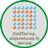# Sequence and SeriesQuantitative Aptitude Questions and Answers section on “Sequence, Series and Progression” with solution and explanation for competitive examinations such as CAT, MBA, SSC, Bank PO, Bank Clerical and other examinations.

# Aptitude Question ID : 94044

The value of * in this sequence $latex 27, 9, 3, *, \frac{1}{3}, \frac{1}{9}, \frac{1}{27}&s=1$ is : [A]-3 [B]-1 [C]1 [D]0 Show Answer 1 The given sequence is based on the following pattern : $latex 27\xrightarrow{\times \frac{1}{3}}9\xrightarrow{\times \frac{1}{3}}3\xrightarrow{\times \frac{1}{3}}\underline1\xrightarrow{\times \frac{1}{3}}\frac{1}{3}\xrightarrow{\times \frac{1}{3}}\frac{1}{9}\xrightarrow{\times \frac{1}{3}}\frac{1}{27}&s=1$ ∴ The value of * is 1, hence option [B] is the right ..

# The next number of the sequence 2, 6, 12, 20, 30, 42, 56, __ is :

The next number of the sequence 2, 6, 12, 20, 30, 42, 56, __ is : [A]60 [B]64 [C]70 [D]72 Show Answer 72 The given sequence is based on the following pattern : $latex 2\xrightarrow{+4}6\xrightarrow{+6}12\xrightarrow{+8}20\xrightarrow{+10}30\xrightarrow{+12}42\xrightarrow{+14}56\xrightarrow{+16}\underline{72}$ ∴ Required number = 72.

# Given below is a finite sequence of numbers with an unknown x : 0, 1, 1, 2, 3, 5, 8, 13, x, 34 the value of x is :

Given below is a finite sequence of numbers with an unknown x : 0, 1, 1, 2, 3, 5, 8, 13, x, 34 the value of x is : [A]17 [B]19 [C]20 [D]21 Show Answer 21 In the given sequence, (starting from the third number) the succeding number is sum of two just precceding numbers. ..

# The sixth term of the sequence 11, 13, 17, 19, 23, __, 29 is :

The sixth term of the sequence 11, 13, 17, 19, 23, __, 29 is : [A]19 [B]22 [C]24 [D]25 Show Answer 25 The sequence is based on the following rule : $latex 11\xrightarrow{+2}13\xrightarrow{+4}17\xrightarrow{+2}19\xrightarrow{+4}23\xrightarrow{+2}\underline{25}\xrightarrow{+4}29$ Hence, the sixth term is 25.

# The wrong term in the sequence 7, 28, 63, 124, 215, 342, 511 is :

The wrong term in the sequence 7, 28, 63, 124, 215, 342, 511 is : [A]28 [B]7 [C]215 [D]124 Show Answer 28 The given sequence is based on the following pattern : $latex 2^{3}-1 = 7$ $latex 3^{3}-1 = \underline{26} , 28(not)$ $latex 4^{3}-1 = 63$ $latex 5^{3}-1 = 125-1 = 124$ \$latex 6^{3}-1 = ..